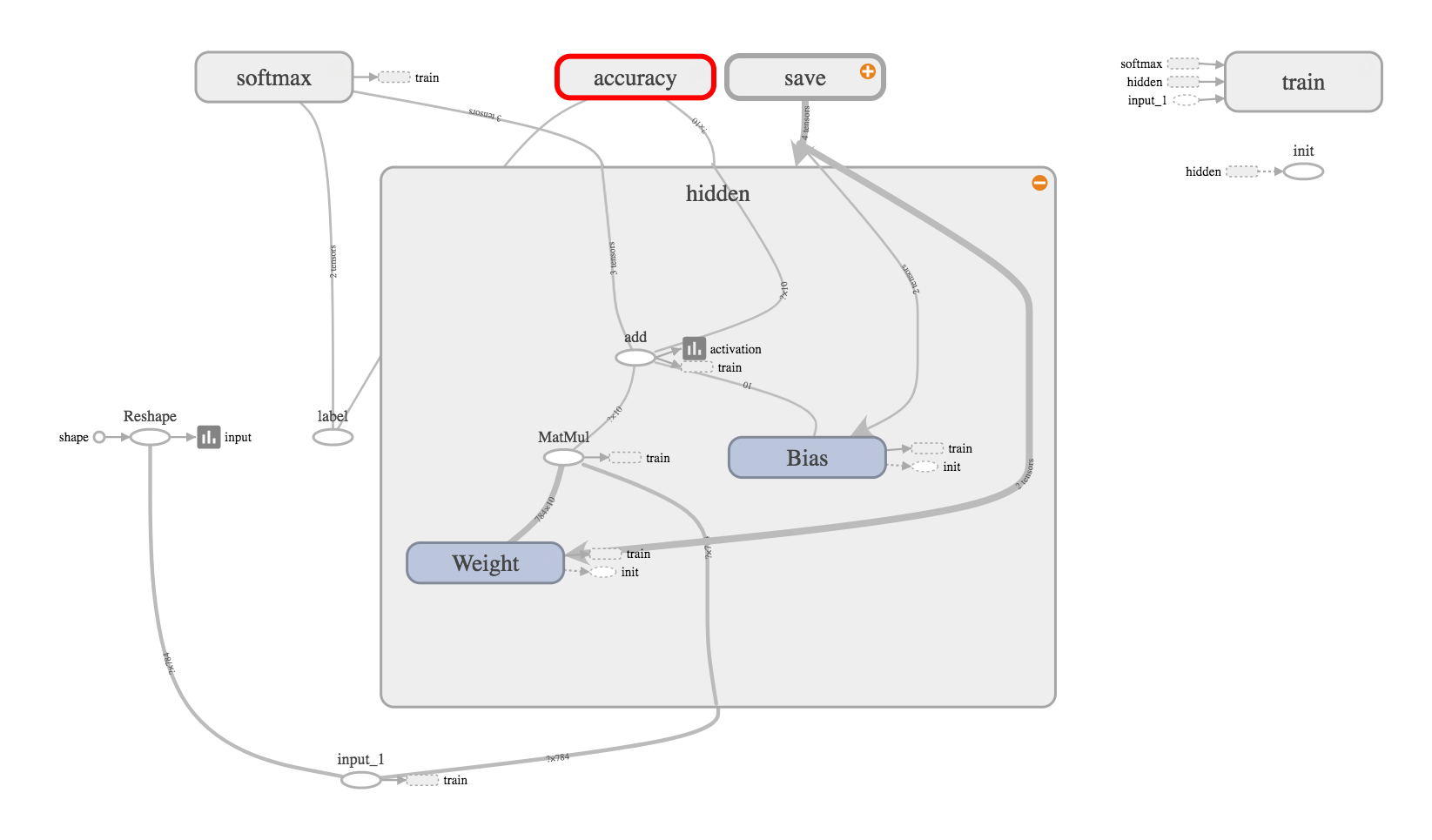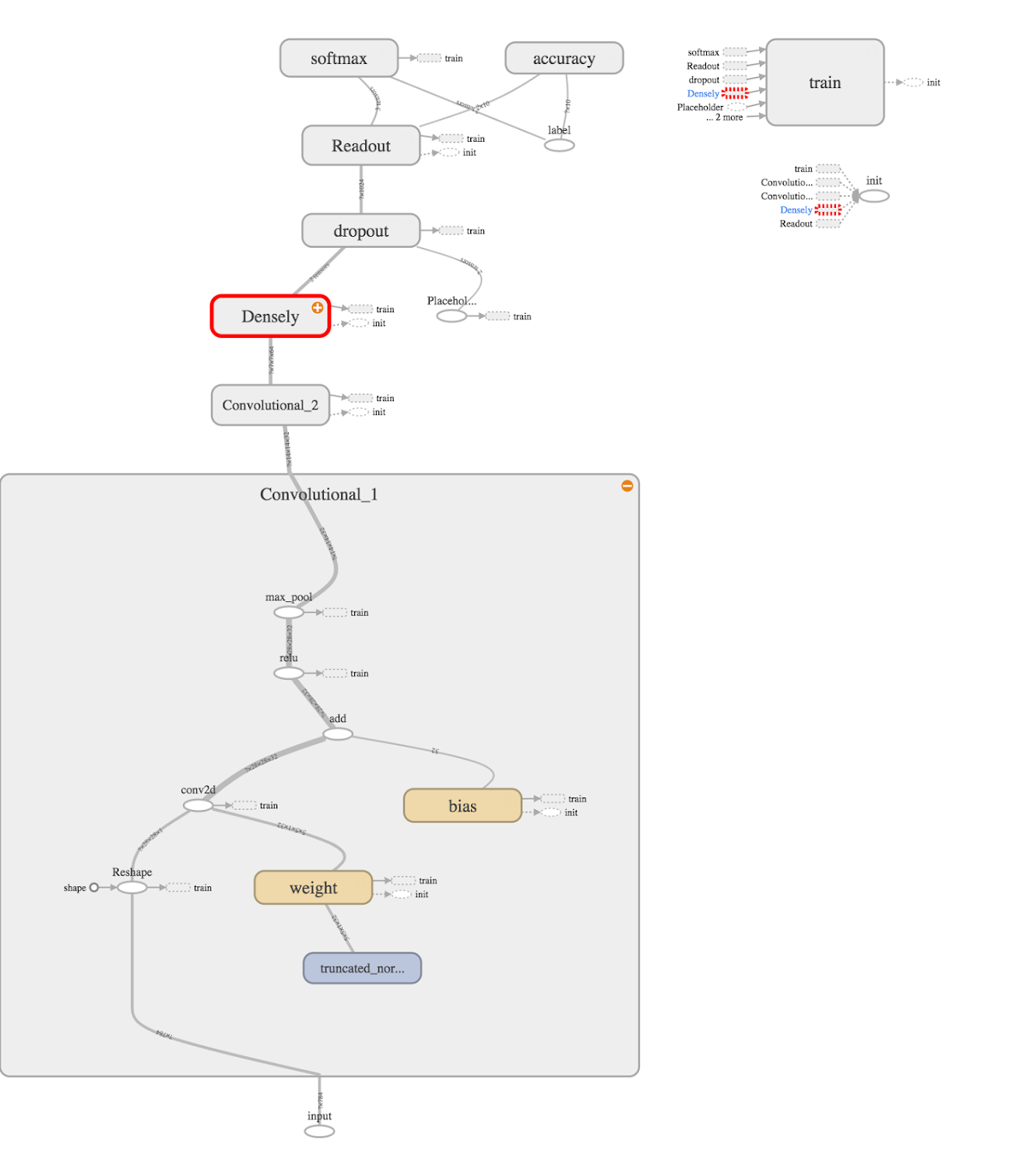[TOC]

# Trailer

Predicting the long-term future is very difficult. Nobody can really do it. The greedy algorithm takes whatever’s working best now and assume the future’s going to be like that forever.
TensorFlow is an open source API for deep learning, developed by Google brain and released on 2015-11-9.
TensorFlow computation is done in a structure called graphs. The inner core of TensorFlow is actually written in C++ to speed up the calculation.
Major improvements in machine learning research result from advances in learning algorithms, computer hardware and high-quality training datasets. MNIST(Mixed National Institute of Standards and Technology) is a large database of handwritten digits for training image processing systems. It contains 60 k training images and 10 k testing images. Currently, the lowest error rate is 0.23% by a hierarchical system of convolutional neural networks.
Here is an interesting animation to illustrate the data flow in neural network: http://playground.tensorflow.org/

# Basic Usage

TensorFlow is a programming system in which you represent computations as graphs. Nodes in the graph are called ops (short for operations). An op takes `Tensors` to performs some computation, where a `Tensor` is a typed multi-dimensional array.
A TensorFlow graph is a description of computations. To compute anything, a graph must be launched in a `Session`. A `Session` places the graph ops onto `Devices`, such as CPUs or GPUs, and provides methods to execute them. These methods return tensors produced by ops as numpy `ndarray` objects in Python.
TensorFlow programs are usually structured into 2 phases:
1. construction phase, that assembles a graph,
2. execution phase, that uses a session to execute ops in the graph.e.g. `tf.Session().run()`
Note: due to imcompatibility, turn on gpu will lead to painful error, so don’t do this: `with tf.device("/cpu:0")`

### interactive session

Usually, we use `Session.run()` method to execute operations.
But in python, we can use `InteractiveSession` class, and the `Tensor.eval()` and `Operation.run()` methods. This avoids having to keep a variable holding the session.

### fetch and feed

usually, we use fetch, where the value is preload. Otherwise, it is feed. we use `placeholder()` for creating ops, then use `feed_dict` to specific the values when we actually run.

# Introduction

• an example of how tensorflow solve a linear regression problem.
• The trick is to initialize the weight and bias in a reasonable value. The network doesn’t have the magic bullet to get arbitrary things right.
• the construction phase is actually writing symbolic math equation. Once I understand, it’s super easy to use.
``````# define weight,bias
W = tf.Variable(tf.random_uniform(, -1.0, 1.0))
b = tf.Variable(tf.zeros())
y_pred = W * X_train + b
# define loss, optimizer
loss = tf.reduce_mean(tf.square(y_pred - y_train))
train_step = optimizer.minimize(loss)
# initialize the variables, build Session
init = tf.global_variables_initializer()
with tf.Session() as sess:
sess.run(init)
for step in range(101):
sess.run(train_step)
print(W.eval(),b.eval())
``````
note:
• train and init are Operations, they can only be `run()`
• W and b are Variable, they can be `run()` or `eval()`

# Beginners

Data extraction and preprocessing are done in `input_data.py`. But that file is just a transfer center. Real work is done `from tensorflow.contrib.learn.python.learn.datasets.mnist import read_data_sets`. Just in case you are interested the data source.
This is a 2-layer(784,10) neural network. Note that the loss function is `tf.nn.softmax_cross_entropy_with_logits(logits=y,labels=y_)`.WARNING: This op expects unscaled logits, since it performs a `softmax` on `logits`internally for efficiency. Do not call this op with the output of `softmax`, as it will produce incorrect results.
batch size =100, gradient descent, learning rate = 0.5, accuracy is 92%.
Actually, I recommend beginner to learn tensorboard at the first place. see here# Experts

layer name size neuron type
input 784
h_pool1 32 conv+relu+max_pool
h_pool2 64 conv+relu+max_pool
h_fc1_drop 1024 relu+dropout
y_conv 10 logits
loss still use `tf.nn.softmax_cross_entropy_with_logits`
optimization use`AdamOptimizer(1e-4)`# Mechanics 101

The main file is `fully_connected_feed.py`,in which the graph is built by `mnist.py`.
It’s 4 layer network (784,128,32,10), the 2 hidden layers being ReLU. It seems complicated than the ‘experts’ version just because it tries to formulate every step, especially the `interference()` that define the graph structure.
The precision is only about 90% in 2000 steps 100 batch size, worse than the beginner version of 92% in 1000 steps 100 batch size. Why it behavior so poorly? It’s partially because the default learning rate is 0.01. I get 96% once I increase the learning rate to 0.1, and 97% when 0.2.
An useful trick learned is to print the loss, which is more directly than the precision. Although such loss is only for a small batch of data, yet this is what exactly happenning during the training process.